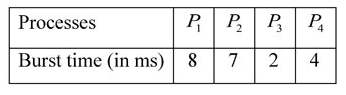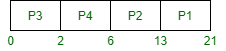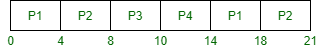# GATE | GATE CS 2020 | Question 60

Consider the following set of processes, assumed to have arrived at time 0. Consider the CPU scheduling algorithms Shortest Job First (SJF) and Round Robin (RR). For RR, assume that the processes are scheduled in the order P1, P2, P3, P4.If the time quantum for RR is 4 ms, then the absolute value of the difference between the average turnaround times (in ms) of SJF and RR (round off to 2 decimal places) is _________ .

Note – This question was Numerical Type.
(A) 5.0
(B) 5.25
(C) 5.50
(D) 5.75

Explanation: According to Shortest Job First (or SJF) CPU Scheduling, gantt chart is,Therefore, Average Turn Around Time (TAT) is,

```= {(21 – 0) + (13 – 0) + (2 – 0) + (6 – 0)} / 4
= 10.5 ```

Now, according to Round Robin (RR) CPU Scheduling with time quantam 4, gantt chart is,Therefore, Average Turn Around Time (TAT) is,

```= {(18 – 0) + (21 – 0) + (10 – 0) + (14 – 0)} / 4
= 15.75```

Hence,

```= ⏐SJF (TAT) – RR(TAT)⏐
= ⏐10.5 – 15.75⏐
= 5.25 ```

Option (B) is correct.

My Personal Notes arrow_drop_up
Article Tags :

1

Please write to us at contribute@geeksforgeeks.org to report any issue with the above content.